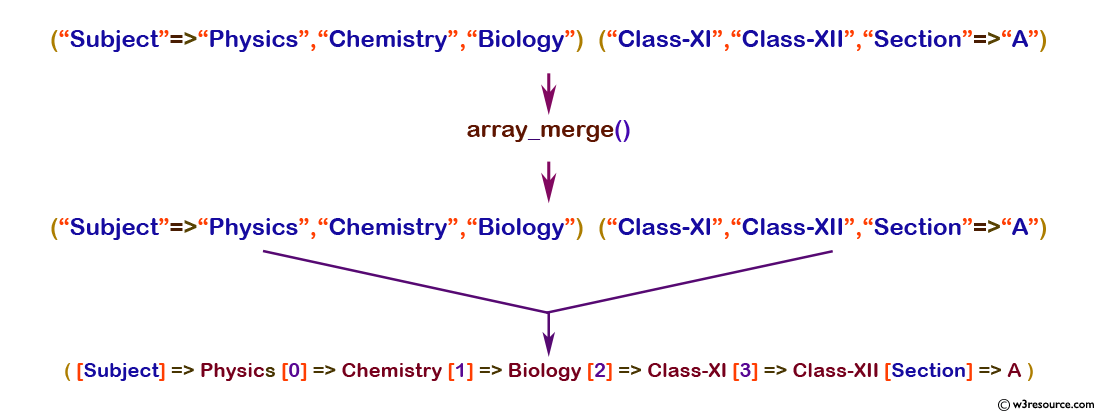# PHP: array_merge() function

## PHP: Merge one or more arrays

The array_merge() function used to merge one or more arrays.
If the input arrays have matching string keys, then the later value will override it's the previous counterpart.
If the input arrays contain numeric keys, the later value will be appended instead of overriding the original value.
If there is only one array, the array is numerically indexed, the keys get reindexed in a continuous way.

Version:

(PHP 4 and above)

Syntax:

`array_merge(array_name1, array_name2, array_name3...) `

Parameters:

Name Description Required /
Optional
Type
array_name1 The name of the array. Required Array
array_name2... The name of the array. Optional Array

Return value:

The merged array.

Value Type: Array

Example:

``````<?php
\$array1=array("Subject" => "Physics","Chemistry", "Biology");
\$array2=array("Class-XI", "Class-XII", "Section"=>"A");
\$result=array_merge(\$array1, \$array2);
print_r(\$result);
?>
``````

Output:

`Array ( [Subject] => Physics  => Chemistry  => Biology  => Class-XI  => Class-XII [Section] => A ) `

Pictorial Presentation:View the example in the browser

Practice here online :

PHP Function Reference

Previous: array_merge_ recursive
Next: array_multisort

﻿

## PHP: Tips of the Day

PHP: Extract numbers from a string

```\$str = 'In My Cart : 11 12 items';
preg_match_all('!\d+!', \$str, \$matches);
print_r(\$matches);
```